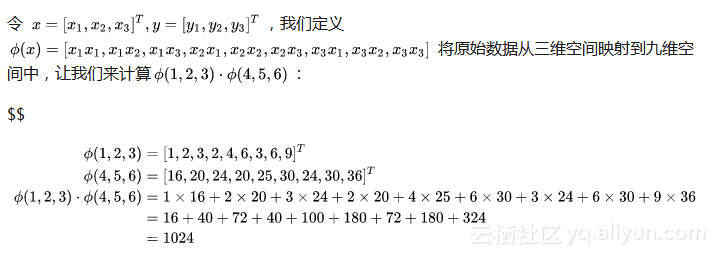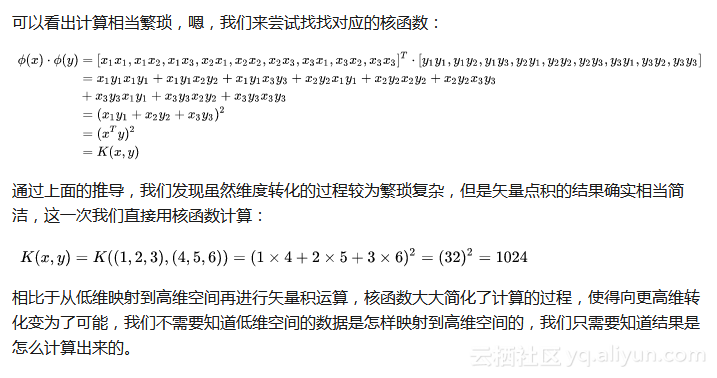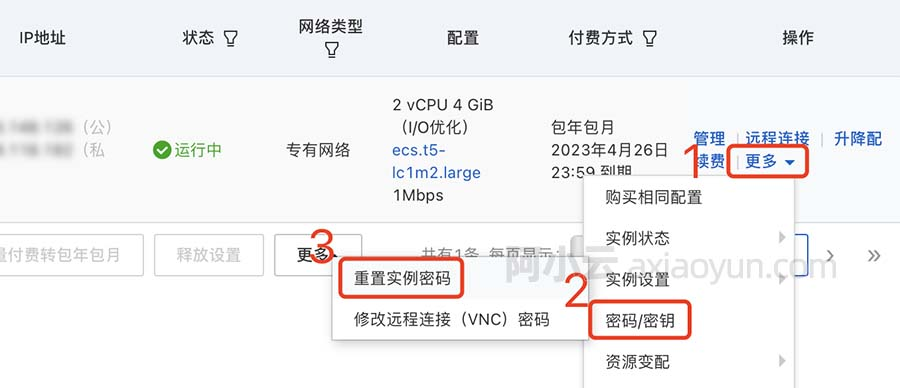# SVM中的核函数

+关注继续查看

1. 回顾多项式回归

$$h_\theta(x_1, x_2) = \theta_0 + \theta_{1}x_1 + \theta_{2}x_{2} + \theta_{3}x_1^{2} + \theta_{4}x_2^{2} + \theta_{5}x_{1}x_2$$

$$h_\theta(x_1, x_2) = \theta_0 + \theta_{1}x_1 + \theta_{2}x_{2} + \theta_{3}x_3 + \theta_{4}x_4 + \theta_{5}x_5$$

2. 核函数的引入

$$\underbrace{ min }_{\alpha} \frac{1}{2}\sum\limits_{i=1,j=1}^{m}\alpha_i\alpha_jy_iy_jx_i \bullet x_j - \sum\limits_{i=1}^{m}\alpha_i \\ s.t. \; \sum\limits_{i=1}^{m}\alpha_iy_i = 0 \\ 0 \leq \alpha_i \leq C$$

$$\underbrace{ min }_{\alpha} \frac{1}{2}\sum\limits_{i=1,j=1}^{m}\alpha_i\alpha_jy_iy_j\phi(x_i) \bullet \phi(x_j) - \sum\limits_{i=1}^{m}\alpha_i \\ s.t. \; \sum\limits_{i=1}^{m}\alpha_iy_i = 0 \\ 0 \leq \alpha_i \leq C$$

$$K(x, z) = \phi(x_i) \bullet \phi(x_j)$$

3. 核函数的介绍

3.1 线性核函数

$$K(x, z) = x \bullet z$$

3.2 多项式核函数

$$K(x, z) = （\gamma x \bullet z + r)^d$$

3.3 高斯核函数

$$K(x, z) = exp(-\gamma||x-z||^2)$$

3.4 Sigmoid核函数
Sigmoid核函数（Sigmoid Kernel）也是线性不可分SVM常用的核函数之一，表达式为：

$$K(x, z) = tanh（\gamma x \bullet z + r)$$9318 06838 02786 04398 0Xshell使用SSH远程登录阿里云ECS服务器CentOS7
10121 07735 0windows server 2008阿里云ECS服务器安全设置

5409 09407 02121 02025 0+关注
61

0

《SaaS模式云原生数据仓库应用场景实践》

《看见新力量：二》电子书